## Friday, 2 March 2012

### M2: Further application of vectors on Euclidean Geometry II

Dot product is a bridge between vector and segment length. When ordinary length calculation does not work, we may rely on position vector to deal with the problems. More importantly we can freely switch between the calculation of dot product of vector and length, while both of them obeys most arithmetic law so that the calculation is convinent.
Besides length, dot product can be used to calculate angle between two vectors, and in the case when we lack information we can still show that two lines are perpendicular.
Example 4. In a convex quadrilateral ABCD where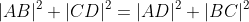, show that AC is perpendicular to BD.

In Euclidean geometry method, we can deal it with simple sine law across the angle between intersection of AC and BD.
In vector method, all we have to do is to show that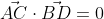Set origin at A. Let the position vector to B,C,D be B,C,D with length b,c,d respectively.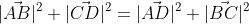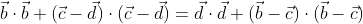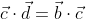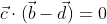Therefore AC is perpendicular to BD.

Sometimes we need to specifically use the basic vector i and j to solve a problem in a more easily way, this is somehow a mixed method between co-geom and vector.
Example 5. For triangle ABC, construct two squares along side AB and BC. Suppose the squares have center K and L respectively. Show that 1) DK is perpendicular to DL; 2) DK=DL.

We let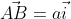,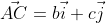.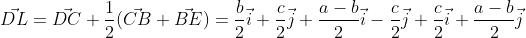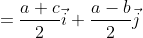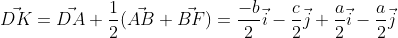Then it's trivial enough to show that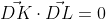and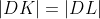. The significance of using i, j is because we can do some rotation of vectors easily instead of multiplying a rotational matrix which is a complicated way.

Example. 6 Show that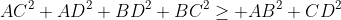(USAMO 1975)

Solutions from mathematical excalibur:
Set origin at A and the vector AB,AC,AD is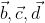.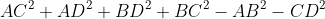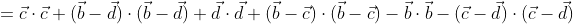And this can be re-grouped into: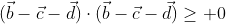Q.E.D.

(we just skip the equality case here)

For problems concerning circles and center, we try to relates position of centers by vectors.
Set circumcenter as the origin.
Centroid: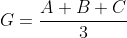which is trivial.
Orthocenter: By Euler line (we are not going to discuss this one),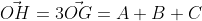.
Incenter: by extention of the angle bisector theorem proved above we have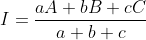.

Example. 7 Let A,B,C be points on a circle, D be the mid-point of AB. Let E be centroid of ACD, show that CD is perpendicular to OE iff AB=AC.

Set origin at O (circumcenter). We have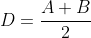,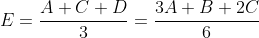,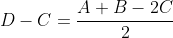CD perpendicular to OE iff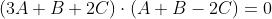, and we know that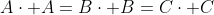, so it's equivalent to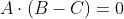, i.e., OA perpendicular to BC, so AB = AC.

Reference: Mathematical Excalibur, Vol #6 No. 5 Vector Geometry

Problems:
1) Let A,B,C,D be points on a circle with center O. AC and BD intersect at T. Show that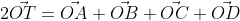.
2) Assume the similar trianlge pair ABC ~ A'B'C', show that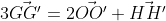(Calin popa)
3) Triangle ABC has no equal side, let G, I, H be its centroid, incenter and orthocenter respectively. Show that angle GIH is larger than 90 degrees. (IMO 1990 unused)